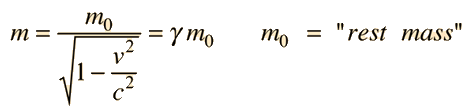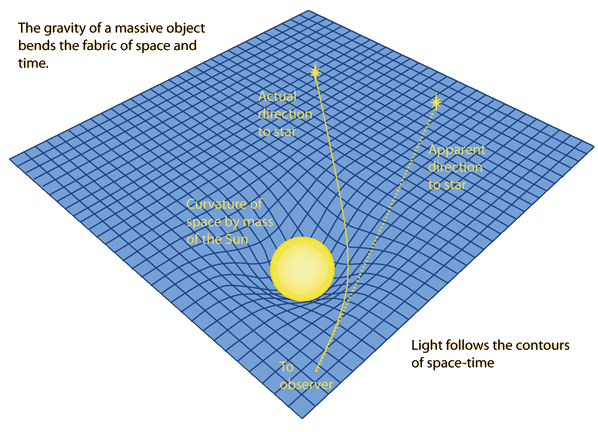# What evidence suggests that the photon has zero mass?

The photon is the quantum of light or other electromagnetic radiation. It is also the exchange particle for the electromagnetic force. It has finite momentum, can be deflected by a gravity field, and can exert a force. So how can we say that it has zero mass?

The first thing to establish is that the term mass is properly limited to what is sometimes called "rest mass", and at least Einstein thought that this was the only proper use for the term mass. The term "relativistic mass" is often used, but it is at best a term that includes not just the mass but some measure of the kinetic energy of a particle with respect to the frame of measurement.

## Evidence for zero mass:

### 1. Non-zero mass implies infinite "relativistic mass".The relativistic mass expression gives a zero in the denominator if the velocity is exactly c, which of course it is for a photon. So a nonzero m0 in the numerator gives an infinite mass.

### 2. Measurements of electromagnetic force imply an infinite range for the force.

The range of an exchange force depends inversely upon the mass of the exchange particle. The nuclear strong force and weak force have very short ranges because of the mass of the exchange particles. The electromagnetic and gravity forces are precisely inverse square law forces with the implication of infinite range. This implies a zero mass exchange particle.

### 3. Light deflection is consistent with the bending of space by gravity.Einstein's famous prediction and the experimental verification of the bending of starlight around the Sun would seem to prove that light has mass and is therefore attracted by the gravitational mass of the Sun. But general relativity indicates that the presence of a gravitational mass bends space in its vicinity, and the accepted interpretation of this observation is that light follows space and therefore follows the curvature of space near the Sun.

 Table of lepton properties
Index

Particle concepts

 HyperPhysics***** Quantum Physics R Nave
Go Back